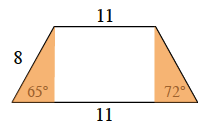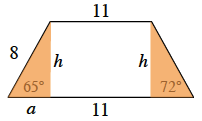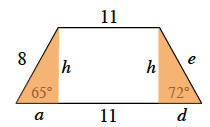Home > GC > Chapter 6 > Lesson 6.2.3 > Problem6-63

6-63.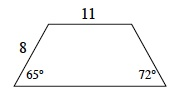Find the area and perimeter of the trapezoid at right. Homework Help ✎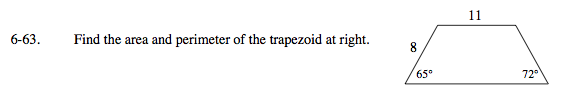Separate the trapezoid into 3 separate figures, including two right triangles and one rectangle.

Solve for a and h.

$\text{sin}(65^{\circ})=\frac{h}{8}\text{ and cos}(65^{\circ})=\frac{a}{8}$

Solve for d and e.

Refer to the Math Notes box in Lesson 5.1.2 if you need help selecting which trigonometric ratio to use when solving for d and e.

Area ≈ 100.55 units²
Perimeter ≈ 43.36 units